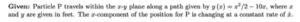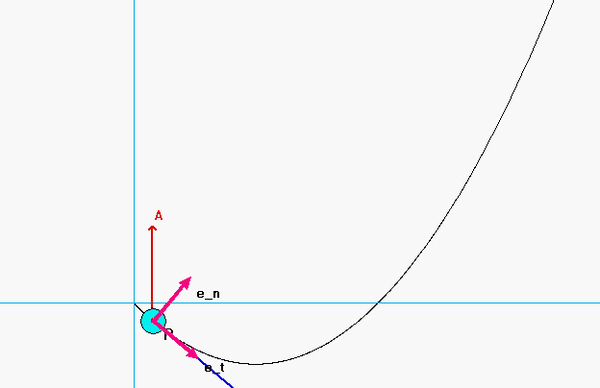# Homework H1.G - Sp23

 Problem statement Solution videoYou can ask questions or answer questions of others here. You can learn from either.

DISCUSSION
Shown in the animation below is the path taken by Particle P.

• As expected the unit vectors et and en are tangent and normal to the path of P, repectively.
• Also seen there is that the velocity vector is always aligned with the tangent unit vector, et, since v = v et.
• The acceleration vector, in general, has both tangential and normal components of acceleration. The normal component always points in the same direction as the unit normal vector, en. The acceleration vector either points "forward" of the motion (for which the rate of change of speed is positive; i.e., increasing is speed), or points "backward" of the motion (for which the rate of change of speed is negative; i.e., slowing down).HINTS
Recall that since you do not know the y-component of motion of P explicitly in terms of time, you will need to use the chain rule to find dy/dt.

It is most convenient to determine the rate of change of speed through the vector projection of the acceleration vector, a, onto the unit tangent vector, et. How do you find the tangent unit vector? Simply divide the velocity vector by its magnitude!   etv/v .

Once you know the rate of change of speed, you can find the radius of curvature ρ through the magnitude of the acceleration.

## 26 thoughts on “Homework H1.G - Sp23”

1.Trisha Boodhoo says:

Did anyone else find that acceleration didn't have a tangential component and velocity didn't have an er vector? Since both these unit vectors must be perpendicular it would make sense but, I am concerned that this may be due to improper differentiation.

1.Trisha Boodhoo says:

Please disregard - this was meant as a comment for 1.E

2.Idris Ismail Muhammad says:

Are the velocity and acceleration for part b supposed to be in cartesian or path description?

1.CMK says:

Beings that all of your given information is in Cartesian, providing the answers in Cartesian would make sense.

2.Kailin Yang says:

part b is cartesian.
part d is path description,
btw: It is most convenient to determine the rate of change of speed through the vector projection of the acceleration vector, a, onto the unit tangent vector, et. How do you find the tangent unit vector? Simply divide the velocity vector by its magnitude! et = v/v .

Once you know the rate of change of speed, you can find the radius of curvature ρ through the magnitude of the acceleration.

1.Benjamin Carl Wassgren says:

We are only asked to find the rate of change of velocity (v dot) in part D, I believe that we do not need to find the radius of curvature.

3.adaetz says:

My sketch of the path of P has the vertex of the quadratic function in the third quadrant of the XY-plane. However, the animation/visualization represents the vertex in the 4th quadrant. Is the animation just for general reference and not exactly representative of the path we are given?

1.Melissa Jewel Brock says:

If you graph the given equation in Desmos, you'll see that the vertex should be in the 4th quadrant.

4.William Jackson Baun says:

When they ask for rate of change of speed how is that different than finding the acceleration that we find in part b?

1.Melissa Jewel Brock says:

I think that means they want you to find the tangential acceleration.

2.Alex L says:

I think the problem statement saying "find rate of change of speed" is just trying to tell you to find path components for acceleration.

3.Kailin Yang says:

It is most convenient to determine the rate of change of speed through the vector projection of the acceleration vector, a, onto the unit tangent vector, et. How do you find the tangent unit vector? Simply divide the velocity vector by its magnitude! et = v/v .

Once you know the rate of change of speed, you can find the radius of curvature ρ through the magnitude of the acceleration.

5.James VanDrunen says:

Is there any specific reason why the value for x is given?

1.Sebastian Magers says:

I believe since P's velocity and acceleration have a y-component as well, they gave us x and x_dot as our parameters to solve for y, y_dot, and y_dot_dot numerically.

6.Allie says:

I think I might be on the right track with this, but I'm not sure. For part B, do we need to use the equation for the radius of curvature at x = 12 in order to find the acceleration? But then using the equation a = v_dot * e_t + (v^2/p) * e_n I am lost as to how to find v_dot. With the way I solved for v it doesn't seem possible.

1.Samuel Elliot Greenaway says:

I don't think you need to use the equation for radius of curvature. You can solve it like that, but I think doing it in cartesian would be easier.

7.Jacob Russell Bunton says:

The rate of change of speed is v_dot right? Then v_dot = e_t * a right?

8.Madeline B says:

I think I am getting thrown off by units. For y_dot, I have (feet - no unit) * ft/s but I know it is suppose to be ft/s. Can I just drop the feet unit?

For y_dot_dot, I have 0 + ft/s when it should be ft/s^2. I am not sure if I can drop units and just do the math then add them back or not.

1.Madeline B says:

I think it's the derivatives messing me up. I keep wanting to use the derivative of x as 1. Will the derivative of x always be x_dot in this class? Even still, my units are messed up.

1.Elijah Allan Collins says:

x_dot is a constant. This is the derivation of x with respect to t

2.Luke Johnson says:

I am also finding that my units for y_dot are ft*ft/s. I know this isn't right but I'm not sure what I am doing wrong.

3.CMK says:

The numeric coefficients in the expression for y(x) have units embedded in them. In the end, x and y both have units of feet.

9.Elijah Allan Collins says:

When doing part D for this problem. Is anyone ending up with clean round numbers or are you getting answers with decimal places? I am not sure if I messed up somewhere.

1.Zachary William Delahunty says:

For part D I got an answer with decimals. I'm pretty sure you use the equation a*et = v(dot).

10.Trenton James Bien says:

For part D, once I have the equation for the Rate of Change of speed of P, do I need to plug in the same point x=12? I would assume so but I just want to make sure

11.Blake Charles Boyer says:

For part D I am using the equation for v_dot from Example 1.C.2. Is that the correct application of this example?